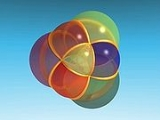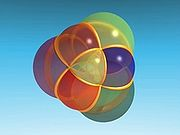xDimensionsEncyclopedia
Dimensions is a French
France
The French Republic , The French Republic , The French Republic , (commonly known as France , is a unitary semi-presidential republic in Western Europe with several overseas territories and islands located on other continents and in the Indian, Pacific, and Atlantic oceans. Metropolitan France...

project that makes educational movies about mathematics
Mathematics
Mathematics is the study of quantity, space, structure, and change. Mathematicians seek out patterns and formulate new conjectures. Mathematicians resolve the truth or falsity of conjectures by mathematical proofs, which are arguments sufficient to convince other mathematicians of their validity...

, focusing on spatial geometry
Euclidean space
In mathematics, Euclidean space is the Euclidean plane and three-dimensional space of Euclidean geometry, as well as the generalizations of these notions to higher dimensions...

. It uses POV-Ray
POV-Ray
The Persistence of Vision Raytracer, or POV-Ray, is a ray tracing program available for a variety of computer platforms. It was originally based on DKBTrace, written by David Kirk Buck and Aaron A. Collins. There are also influences from the earlier Polyray raytracer contributed by its author...

to render some of the animations, and the films are release under a Creative Commons licence.The film is separated in nine chapters, which follow this plot:
• Chapter 1: Dimension two explains Earth
Earth
Earth is the third planet from the Sun, and the densest and fifth-largest of the eight planets in the Solar System. It is also the largest of the Solar System's four terrestrial planets...

's coordinate system, and introduces the stereographic projection
Stereographic projection
The stereographic projection, in geometry, is a particular mapping that projects a sphere onto a plane. The projection is defined on the entire sphere, except at one point — the projection point. Where it is defined, the mapping is smooth and bijective. It is conformal, meaning that it...

.
• Chapter 2: Dimension three discusses how two-dimensional beings would imagine three-dimensional objects.
• Chapters 3 and 4: The fourth dimension talk about four-dimensional polytope
Polytope
In elementary geometry, a polytope is a geometric object with flat sides, which exists in any general number of dimensions. A polygon is a polytope in two dimensions, a polyhedron in three dimensions, and so on in higher dimensions...

s (polychora), projecting the regular ones stereographically on the three-dimensional space.
• Chapters 5 and 6: Complex numbers are about the square root of negative numbers
Complex number
A complex number is a number consisting of a real part and an imaginary part. Complex numbers extend the idea of the one-dimensional number line to the two-dimensional complex plane by using the number line for the real part and adding a vertical axis to plot the imaginary part...

, transformations, and fractal
Fractal
A fractal has been defined as "a rough or fragmented geometric shape that can be split into parts, each of which is a reduced-size copy of the whole," a property called self-similarity...

s.
• Chapters 7 and 8: Fibration show what a fibration
Fibration
In topology, a branch of mathematics, a fibration is a generalization of the notion of a fiber bundle. A fiber bundle makes precise the idea of one topological space being "parameterized" by another topological space . A fibration is like a fiber bundle, except that the fibers need not be the same...

is. Complex numbers are used again, and there are circle
Circle
A circle is a simple shape of Euclidean geometry consisting of those points in a plane that are a given distance from a given point, the centre. The distance between any of the points and the centre is called the radius....

s and tori
Torus
In geometry, a torus is a surface of revolution generated by revolving a circle in three dimensional space about an axis coplanar with the circle...

rotating and being transformed.
• Chapter 9: Proof emphasizes the importance of proofs
Mathematical proof
In mathematics, a proof is a convincing demonstration that some mathematical statement is necessarily true. Proofs are obtained from deductive reasoning, rather than from inductive or empirical arguments. That is, a proof must demonstrate that a statement is true in all cases, without a single...

in mathematics, and proves the circle-conservationess of the stereographic projection as an example.